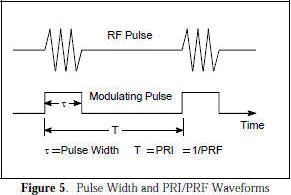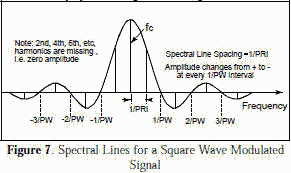Please Support RF Cafe by purchasing my  ridiculously low−priced products, all of which I created.

RF Workbench  (shareware)# Electronic Warfare and Radar Systems Engineering Handbook - Modulation -

MODULATION

Modulation is the process whereby some characteristic of one wave is varied in accordance with some characteristic of another wave. The basic types of modulation are angular modulation (including the special cases of phase and frequency modulation) and amplitude modulation. In missile radars, it is common practice to amplitude modulate the transmitted RF carrier wave of tracking and guidance transmitters by using a pulsed wave for modulating, and to frequency modulate the transmitted RF carrier wave of illuminator transmitters by using a sine wave.Frequency Modulation (FM) - As shown in Figure 1, an unmodulated RF signal in the time domain has only a single spectral line at the carrier frequency (fc) in the frequency domain. If the signal is frequency modulated, as shown in Figure 2, the spectral line will correspondingly shift in the frequency domain.

Amplitude Modulation (AM) - If the signal in Figure 1 is amplitude modulated by a sinewave as shown in Figure 3, sidebands are produced in the frequency domain at Fc ± FAM. AM other than by a pure sine wave will cause additional sidebands normally at Fc ± nFAM, where n equals 1, 2, 3, 4, etc.

Pulse modulation is a special case of AM wherein the carrier frequency is gated at a pulsed rate. When the reciprocal of the duty cycle of the AM is a whole number, harmonics corresponding to multiples of that whole number will be missing, e.g. in a 33.33% duty cycle, AM wave will miss the 3rd, 6th, 9th, etc. harmonics, while a square wave or 50% duty cycle triangular wave will miss the 2nd, 4th, 6th, etc. harmonic, as shown in Figure 4. It has sidebands in the frequency domain at Fc ± nFAM, where n = 1, 3, 5, etc. The amplitude of the power level follows a sine x / x type distribution.Figure 5 shows the pulse width (PW) in the time domain which defines the lobe width in the frequency domain (Figure 6). The width of the main lobe is 2/PW, whereas the width of a side lobe is 1/PW. Figure 5 also shows the pulse repetition interval (PRI) or its reciprocal, pulse repetition frequency (PRF), in the time domain. In the frequency domain, the spectral lines inside the lobes are separated by the PRF or 1/PRI, as shown in Figures 7 and 8. Note that Figures 7 and 8 show actual magnitude of the side lobes, whereas in Figure 4 and 6, the absolute value is shown.

The magnitude of each spectral component for a rectangular pulse can be determined from the following formula:Figure 7 shows the spectral lines for a square wave (50% duty cycle), while Figure 8 shows the spectral lines for a 33.33% duty cycle rectangular wave signal.Figure 9 shows that for square wave AM, a significant portion of the component modulation is contained in the first few harmonics which comprise the wave. There are twice as many sidebands or spectral lines as there are harmonics (one on the plus and one on the minus side of the carrier). Each sideband represents a sine wave at a frequency equal to the difference between the spectral line and fc .

A figure similar to Figure 9 can be created for any rectangular wave. The relative amplitude of the time domain sine wave components are computed using equation . Each is constructed such that at the midpoint of the pulse the sine wave passes through a maximum (or minimum if the coefficient is negative) at the same time. It should be noted that the "first" harmonic created using this formula is NOT the carrier frequency, fc , of the modulated signal, but at Fc ± FAM.

While equation  is for rectangular waves only, similar equations can be constructed using Fourier coefficients for other waveforms, such as triangular, sawtooth, half sine, trapezoidal, and other repetitive geometric shapes.

PRI Effects - If the PW remains constant but PRI increases, the number of sidelobes remains the same, but the number of spectral lines gets denser (move closer together) and vice versa (compare Figure 7 and 8). The spacing between the spectral lines remains constant with constant PRI.

Pulse Width (PW) Effects - If the PRI remains constant, but the PW increases, then the lobe width decreases and vice versa. If the PW approaches PRI, the spectrum will approach "one lobe", i.e., a single spectral line. The spacing of the lobes remains constant with constant PW.

RF Measurements - If the receiver bandwidth is smaller than the PRF, the receiver will respond to one spectral line at a time. If the receiver bandwidth is wider than the PRF but narrower than the reciprocal of the PW, the receiver will respond to one spectral envelope at a time.

Jet Engine Modulation (JEM)Section 2-6 addresses the Doppler shift in a transmitted radar signal caused by a moving target. The amount of Doppler shift is a function of radar carrier frequency and the speed of the radar and target. Moving or rotating surfaces on the target will have the same Doppler shift as the target, but will also impose AM on the Doppler shifted return (see Figure 10).

Reflections off rotating jet engine compressor blades, aircraft propellers, ram air turbine (RAT) propellers used to power aircraft pods, helicopter rotor blades, and protruding surfaces of automobile hubcaps will all provide a chopped reflection of the impinging signal. The reflections are characterized by both positive and negative Doppler sidebands corresponding to the blades moving toward and away from the radar respectively.

Therefore, forward/aft JEM doesn't vary with radar carrier frequency, but the harmonics contained in the sidebands are a function of the PRF of the blade chopping action and its amplitude is target aspect dependent, i.e. blade angle, intake/exhaust internal reflection, and jet engine cowling all effect lateral return from the side. If the aspect angle is too far from head-on or tail-on and the engine cowling provides shielding for the jet engine, there may not be any JEM to detect. On the other hand, JEM increases when you are orthogonal (at a right angle) to the axis of blade rotation. Consequently for a fully exposed blade as in a propeller driven aircraft or helicopter, JEM increases with angle off the boresight axis of the prop/rotor.